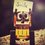# Charged Spheres

Consider two identical spheres A and B with charge Q each. Now a third identical neutral sphere C is touched with A then B then A then B ane so on .

Will the process terminate, I mean will there be a time when there is no charge redistribution.Note by Kushal Patankar
4 years, 9 months ago

This discussion board is a place to discuss our Daily Challenges and the math and science related to those challenges. Explanations are more than just a solution — they should explain the steps and thinking strategies that you used to obtain the solution. Comments should further the discussion of math and science.

When posting on Brilliant:

• Use the emojis to react to an explanation, whether you're congratulating a job well done , or just really confused .
• Ask specific questions about the challenge or the steps in somebody's explanation. Well-posed questions can add a lot to the discussion, but posting "I don't understand!" doesn't help anyone.
• Try to contribute something new to the discussion, whether it is an extension, generalization or other idea related to the challenge.

MarkdownAppears as
*italics* or _italics_ italics
**bold** or __bold__ bold
- bulleted- list
• bulleted
• list
1. numbered2. list
1. numbered
2. list
Note: you must add a full line of space before and after lists for them to show up correctly
paragraph 1paragraph 2

paragraph 1

paragraph 2

[example link](https://brilliant.org)example link
> This is a quote
This is a quote
    # I indented these lines
# 4 spaces, and now they show
# up as a code block.

print "hello world"
# I indented these lines
# 4 spaces, and now they show
# up as a code block.

print "hello world"
MathAppears as
Remember to wrap math in $$ ... $$ or $ ... $ to ensure proper formatting.
2 \times 3 $2 \times 3$
2^{34} $2^{34}$
a_{i-1} $a_{i-1}$
\frac{2}{3} $\frac{2}{3}$
\sqrt{2} $\sqrt{2}$
\sum_{i=1}^3 $\sum_{i=1}^3$
\sin \theta $\sin \theta$
\boxed{123} $\boxed{123}$

Sort by:

Kushal Patankar

This sort of scenario arises in many places. The answer is that the final charges will be $\frac {2Q} {3}$. There are many ways to explain this, I think it can be explained with a fully qualitative reasoning(no math), but since you have asked for it:

Let us think about it this way, let at some point of time the spheres $S1$ and $S2$ have charges $Q1$ and $Q2$. The sphere we can move is $S3$ and it has a charge $Q3$. When we touch a sphere with the movable one, what happens is that the charges on the spheres become equal. If we touch $S1$, the charge on each sphere becomes $(Q1+Q3)/2$. Consider this series:

$1,0.5,0.75,0.625,0.6875,...$

In the above series, the following rule is applicable for the $n^{th}$ term:

$a_n=(a_{n-1}+a_{n-2})/2$

Observe that this is the series of the values of the charges(assume $Q=1C$) on each sphere after each step starting from before the movable sphere comes into contact with $S2$. Write the successive charges on each sphere after each contact, and you will see that we get the above series.

We define a generating function like below:

$f(x)=a_0+a_1 x+a_2 x^2+ a_3 x^3+a_4 x^4+...$

For the current discussion, we have:$a_0=1,a_1=0.5$.

Try to solve this generating function in order to get a closed form expression for $a_n$. I will leave this for you to do on your own. The answer we get is: $a_n= \frac {2^{n+1}+(-1)^n} {3 \times 2^n}$.

You can now figure out the necessary details when $n \to \infty$

- 4 years, 9 months ago

- 4 years, 9 months ago

Thanks bro. I will do the rest now. Thanks again.

- 4 years, 9 months ago

Initially when A is touched to C, the charge flows unless both have equal charge i.e. Q/2 . Subsequently when C is touched to B, charge flows until both posses equal charge i.e. 3Q/4. Moving further with this trend, there would come a point when the 3 balls posses equal charge I.e. 2Q/3 and there would be no further charge redistribution. I hope you get the point. Please point out my misconceptions if any. :)

- 4 years, 9 months ago

Yes you are right . But can the neutral ball aquire a charge of 2Q/3

If yes then how many touchs are required.

Are they finite .

- 4 years, 9 months ago

I think the touches tend to infinity.

- 4 years, 9 months ago

Can you provide the mathematical explanation for that.

- 4 years, 9 months ago